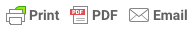### What a p-value tells you about statistical significance

By Saul McLeod, published 2019When you perform a statistical test a p-value helps you determine the significance of your results in relation to the null hypothesis.

The null hypothesis states that there is no relationship between the two variables being studied (one variable does not affect the other). It states the results are due to chance and are not significant in terms of supporting the idea being investigated. Thus, the null hypothesis assumes that whatever you are trying to prove did not happen.

The alternative hypothesis is the one you would believe if the null hypothesis is concluded to be untrue.

The alternative hypothesis states that the independent variable did affect the dependent variable, and the results are significant in terms of supporting the theory being investigated (i.e. not due to chance).

###### How do you know if a p-value is statistically significant?

The level of statistical significance is often expressed as a p-value between 0 and 1. The smaller the p-value, the stronger the evidence that you should reject the null hypothesis.

• A p-value less than 0.05 (typically ≤ 0.05) is statistically significant. It indicates strong evidence against the null hypothesis, as there is less than a 5% probability the null is correct (and the results are random). Therefore, we reject the null hypothesis, and accept the alternative hypothesis.

However, this does not mean that there is a 95% probability that the research hypothesis is true. The p-value is conditional upon the null hypothesis being true is unrelated to the truth or falsity of the research hypothesis.

• A p-value higher than 0.05 (> 0.05) is not statistically significant and indicates strong evidence for the null hypothesis. This means we retain the null hypothesis and reject the alternative hypothesis. You should note that you cannot accept the null hypothesis, we can only reject the null or fail to reject it.

A statistically significant result cannot prove that a research hypothesis is correct (as this implies 100% certainty).

Instead, we may state our results “provide support for” or “give evidence for” our research hypothesis (as there is still a slight probability that the results occurred by chance and the null hypothesis was correct – e.g. less than 5%).

###### How to report a p-value APA style

The 6th edition of the APA style manual (American Psychological Association, 2010) states the following on the topic of reporting p-values:

“When reporting p values, report exact p values (e.g., p = .031) to two or three decimal places. However, report p values less than .001 as p < .001. The tradition of reporting p values in the form p < .10, p < .05, p < .01, and so forth, was appropriate in a time when only limited tables of critical values were available.” (p. 114)

###### Note:

• Do not use 0 before the decimal point for the statistical value p as it cannot equal 1, in other words, write p = .001 instead of p = 0.001.
• Please pay attention to issues of italics (p is always italicized) and spacing (either side of the = sign).
• p = .000 (as outputted by some statistical packages such as SPSS) is impossible and should be written as p < .001.
• The opposite of significant is "nonsignificant", not "insignficant".

###### Why the p-value is not enough

A lower p-value is sometimes interpreted as meaning there is a stronger relationship between two variables. However, statistical significance means that it is unlikely that the null hypothesis is true (less than 5%).

To understand the strength of the difference between two groups (control vs. experimental) a researcher needs to calculate the effect size.

McLeod, S. A. (2019, May 20). What a p-value tells you about statistical significance. Simply Psychology. https://www.simplypsychology.org/p-value.html